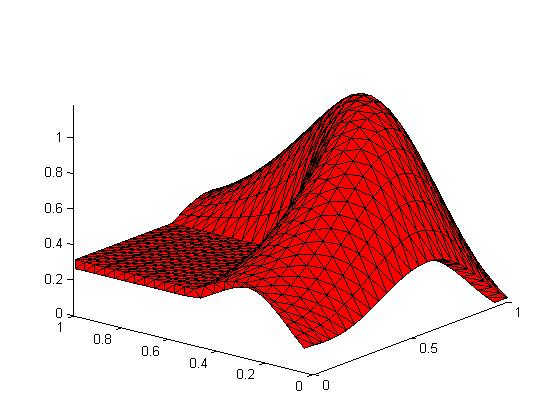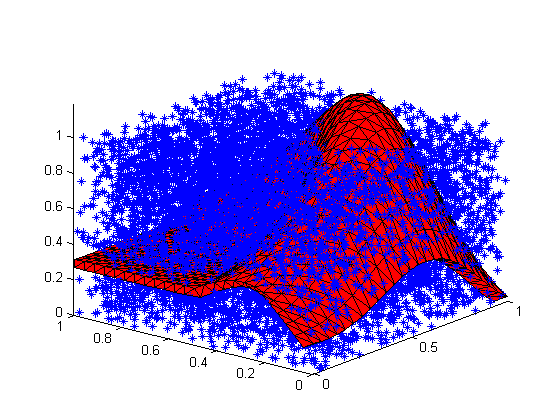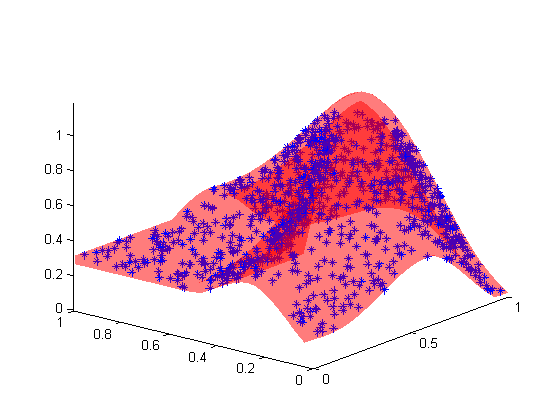# INPOLYHEDRON

Sean's pick this week is inpolyhedron by Sven.

### Polygons, Polyhedra and Convexity

It's often useful to know if a point is inside of a polygon (two dimensions) and sometimes if a point is inside of a polyhedron (three dimensions). For two dimensions, MATLAB ships with inpolygon, a nice function to handle this. However, this same operation in three dimensions becomes more complicated. It is simplified if the object is convex. In this case, the vertices can be represented as a set of constraints and we can apply these constraints to the points to test whether they are inside or not. Matt J does this in his vert2lcon function (The runner-up, who will also get some MathWorks swag, for this week's post!).

But what about when the polydehron is not necessarily convex? Now we no longer have this luxury and have to resort to more complex algorithms.

Fortunately, Sven has done the work for us!

% Load shellVertices, shellfaces from Paul's Blog:

% Draw the membrane:
patch('Vertices',shellVertices,...
'Faces',shellFaces,...
'FaceColor','r');
axis tight
view(-51,24)Add 10000 random points to it

pts = rand([10000,3]); %Generate a random 10000pts
hold on;
plot3(pts(:,1),pts(:,2),pts(:,3),'b*'); %add points to plotFind the points in the polyhedron

in = inpolyhedron(shellFaces,shellVertices,pts,'FlipNormals',true);
delete(hLine); %clean up
set(hPatch,'FaceAlpha',0.3,...
'EdgeColor','none'); %make the points inside visibleWoohoo!